# GSEB Solutions Class 12 Maths Chapter 9 Differential Equations Ex 9.6

Gujarat Board GSEB Textbook Solutions Class 12 Maths Chapter 9 Differential Equations Ex 9.6 Textbook Questions and Answers.

## Gujarat Board Textbook Solutions Class 12 Maths Chapter 9 Differential Equations Ex 9.6

For each of the differential equations given in questions 1 to 12, find the general solution:

Question 1.
$$\frac{dy}{dx}$$ + 2y = sin x
Solution:
$$\frac{dy}{dx}$$ + 2y = sin x
Here, I.F. = e∫22dx = e2x
∴ Solution to the differential equation is
ye2x = ∫(sin x)e2x dx = I1 ………………. (1)
Now, I1 = ∫e2x sin x dx
Integrating by parts, taking e2x as a first function, we get
I1 = e2x(- cos x) – ∫2e2x(- cos x) dx
= – e2x cos x + 2[e2x sin x – ∫2e2x sin x dx]
= – e2x cos x + 2 sin x e2x – 4∫e2x sin x dx
= e2x (2 sin x – cos x) – 4I1
∴ 5I1 = e2x(2 sin x – cos x) + 5C
∴ I1 = $$\frac{e^{2 x}}{5}$$(2 sin x – cos x) + C
Putting the value of I1 in (1), the general solution is
ye2x = $$\frac{e^{2 x}}{5}$$(2 sin x – cos x) + C
or 5y = 2 sin x – cos x + 5Ce-2x.Question 2.
$$\frac{dy}{dx}$$ + 3y = e-2x
Solution:
Here, I.F. = e∫3dx = e3x
∴ Solution is
y e3x = ∫e-2x.e3x dx + C
= ∫exdx + C = ex + C
∴ General Solution is
ye3x = ex + C or y = e-2x + Ce-3x.

Question 3.
$$\frac{dy}{dx}$$ + $$\frac{y}{x}$$ = x2
Solution:
$$\frac{dy}{dx}$$ + $$\frac{y}{x}$$
= x2 or $$\frac{dy}{dx}$$ + $$\frac{1}{x}$$y = x2.
This is a linear equation of the form $$\frac{dy}{dx}$$ + Py = Q
where P = $$\frac{1}{x}$$ and Q = x2.
∴ I.F. = e∫Pdx = $$e^{\int \frac{1}{x} d x}$$ = elogx = x.
∴ Solution is
y × I.F. = ∫Q × I.F. dx + C
⇒ y × x = ∫x2.x dx + C
or xy = $$\frac{x^{4}}{4}$$ + C
⇒ y = $$\frac{x^{3}}{4}$$ + $$\frac{C}{x}$$.Question 4.
$$\frac{dy}{dx}$$ + sec x.y = tan x, (0 ≤ x < $$\frac{π}{2}$$)
Solution:
$$\frac{dy}{dx}$$ + sec x.y = tan x
This is a linear equation of the form $$\frac{dy}{dx}$$ + Py = Q
where P = sec x and Q = tan x.
∴ I.F. = e∫Pdx = e∫secxdx
= elog(secx+tanx) = sec x + tan x.
∴ The solution is
y × I.F. = ∫Q × I.F. dx + C
or y × (sec x + tan x) = ∫tan x(sec x + tan x)dx + C
= ∫sec x tan x dx + ∫tan2 x dx + C.
= sec x + ∫(sec2 x – 1)dx + C (∵ 1 + tan2x = sec2x)
= (sec x + tan x) – x + C
∴ Required solution is
∴ y(sec x + tan x) = (sec x + tan x) – x + C.

Question 5.
cos2x $$\frac{dy}{dx}$$ + y = tan x, (0 ≤ x < $$\frac{π}{2}$$)
Solution:
cos2x$$\frac{dy}{dx}$$ + y = tan x, (0 ≤ x < $$\frac{π}{2}$$)
or $$\frac{dy}{dx}$$ + $$\frac{1}{\cos ^{2} x}$$y = $$\frac{tanx}{\cos ^{2} x}$$
This equation is of the form $$\frac{dy}{dx}$$ + Py = Q, where⇒ Solution of the given equation is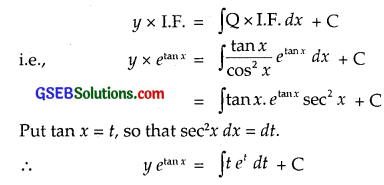Put tan x = t, so that sec2x dx = dt
∴ y etanx = ∫t et dt + C
Integrating by parts, taking t as a first function, we get
y etanx = t et – ∫1.et dt + C
= t et – et + C
= tan x etanx – etanx + C (Putting t = tan x)
or y = tan x – 1 + Ce-tanx.Question 6.
x $$\frac{dy}{dx}$$ + 2y = x2 log x
Solution:
x $$\frac{dy}{dx}$$ + 2y = x2 log x or $$\frac{dy}{dx}$$ + $$\frac{2}{x}$$y = x log x.
This is a linear equation of the form $$\frac{dy}{dx}$$ + Py = Q
where P = $$\frac{2}{x}$$ and Q = x log x.
∴ I.F. = e∫Pdx = $$e^{\int \frac{2}{x} d x}$$ = e2logx.
∴ The solution of the given equation is
y × I.F. = ∫Q × I.F. dx + C
or y × x2 = ∫(x log x)x2 dx + C
= ∫(x3 log x)dx + C
Integrating by parts, taking log x as a first function, we get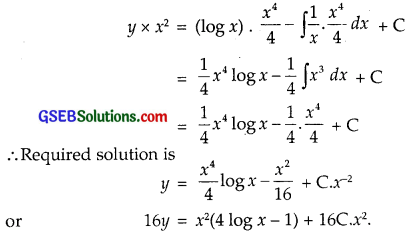Question 7.
x log x $$\frac{dy}{dx}$$ + y = $$\frac{2}{x}$$ log x
Solution:
x log x $$\frac{dy}{dx}$$ + y = $$\frac{2}{x}$$ log x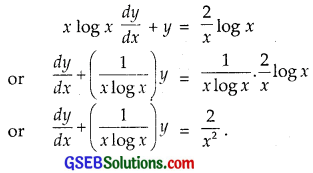This is a linear equation of the form $$\frac{dy}{dx}$$ + Py = QIntegrating by parts, taking log x as first function, we get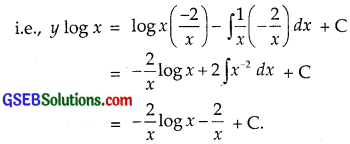∴ Required solution is y log x = – $$\frac{2}{x}$$(1 + log x) + C.Question 8.
(1 + x2)dy + 2xy dx = cot x dx, (x ≠ 0)
Solution:This is a linear equation of the form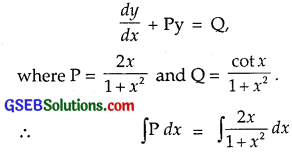Put 1 + x2 = t, so that 2x dx = dt.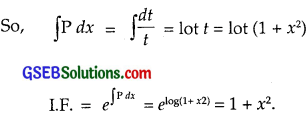Hence the solution is∴ Required solution is
y(1 + x2) = log|sin x| + C.

Question 9.
x $$\frac{dy}{dx}$$ + y – x + xy cot x = 0 (x ≠ 0)
Solution: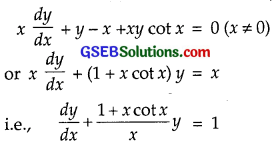This is a linear equation of the form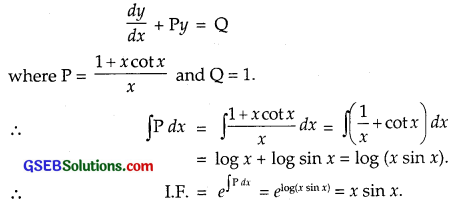Now, the solution is
y × I.F. = ∫Q × I.F. dx + C
y × x sin x = ∫1 × x sin x dx + C
= – x cos x + sin x + C
Thus, the solution is
xy sin x = – x cos x + sin x + C.
or y = – cot x + $$\frac{1}{x}$$ + $$\frac{C}{xsinx}$$.Question 10.
(x + y)$$\frac{dy}{dx}$$ = 1
Solution:
(x + y)$$\frac{dy}{dx}$$ = 1 or $$\frac{dx}{dy}$$ = x + y
or $$\frac{dx}{dy}$$ – x = y
This equation is of the form $$\frac{dx}{dy}$$ + Px = Q
where P = – 1 and Q = y.
So, I.F. = e∫-1.dy = e-y
∴ The solution is
x × I.F. = ∫Q × I.F. dy + C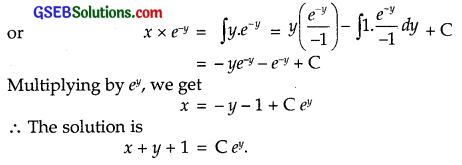Multiplying by ey, we get
x = – y – 1 + C ey
∴ The solution is
x + y + 1 = C ey.

Question 11.
y dx + (x – y2) dy = 0
Solution:
y dx + (x – y2)dy = 0 or y $$\frac{dy}{dx}$$ + x – y2 = 0
or $$\frac{dx}{dy}$$ + $$\frac{1}{y}$$ x = y.
This is a linear equation of the form $$\frac{dx}{dy}$$ + Px = Q
where P = $$\frac{1}{y}$$ and Q = y.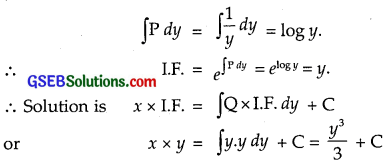Question 12.
(x + 3y2)$$\frac{dy}{dx}$$ = y(y > 0).
Solution: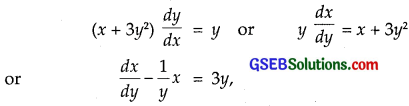which is a linear equation of the form $$\frac{dx}{dy}$$ + Px = Q
where P = – $$\frac{1}{y}$$ and Q = 3y.Hence, the required solution is
x = 3y2 + Cy.

For each of the differential equations given ¡n questions 13 to 15, find particular solution satisfying the given conditions:

Question 13.
$$\frac{dy}{dx}$$ + 2y tan x = sin x; y = 0, when x = $$\frac{π}{3}$$.
Solution:
$$\frac{dy}{dx}$$ + 2y tan x = sin x
This is a linear equation of the form $$\frac{dy}{dx}$$ + Py = Q
where P = 2 tan x and Q = sin x.
∴ ∫P dx = ∫2 tan x dx = 2 log sec x
= log sec2x.
∴ I.F. = e∫Pdx = $$e^{\log \sec ^{2} x}$$ = sec2x.
∴ Solution is y × I.F. = ∫Q × I.F. dx + C
y × sec2x = ∫sin x sec2 x dx + C
= ∫sec x tan x dx + C = sec x + C.
Hence, the required solution is y = cos x + C cos2x.
Now, we have : y = 0, when x = $$\frac{π}{3}$$
∴ 0 = cos $$\frac{π}{3}$$ + C cos2 $$\frac{π}{3}$$
= $$\frac{1}{2}$$ + $$\frac{C}{4}$$
∴ C = – 2.
∴ Required particular solution is y = cos x – 2 cos2x.Question 14.
(1 + x2) $$\frac{dy}{dx}$$ + 2xy = $$\frac{1}{1+x^{2}}$$; y = 0, when x = 1.
Solution:Using y × I.F. = ∫Q × I.F. dx + C, we get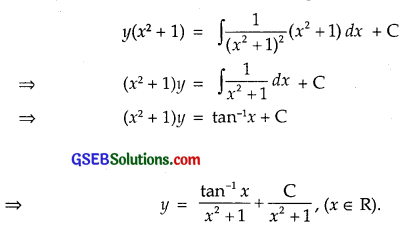We have: y = 0, when x = 1.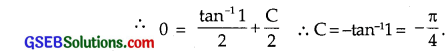Required particular solution isQuestion 15.
$$\frac{dy}{dx}$$ – 3y cot x = sin 2x; y = 2, when x = $$\frac{π}{2}$$.
Solution:
$$\frac{dy}{dx}$$ – 3y cot x
This is a linear equation of the form $$\frac{dy}{dx}$$ + Py = Q
where P = – 3 cot x and Q = sin 2x.
∴ ∫P dx = ∫- 3 log x dx = – 3 log sin x
= log cosec3x.
∴ Integrating Factor = e∫Pdx = $$e^{\log \operatorname{cosec}^{3} x}$$ = cosec3x.
∴ Solution is y × I.F. = ∫Q × I.F. dx + C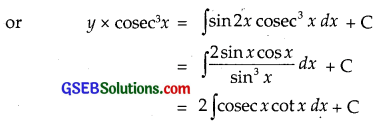= – 2 cosec x + C
or y = – 2 sin2x + C sin3x.
Now, y = 2, when x = $$\frac{π}{2}$$.
∴ 2 = – 2 + C ∴ C = 4
∴ Required particular solution is
y = – 2 sin2x + 4 sin3x
= – 2 sin2x (1 – 2 sin x).Question 16.
Find the equation of the curve passing through the origin,
given that the slope of the tangent to the curve at any point (x, y) is equal to the sum of the coordinates of the point.
Solution:
Let y = f(x) be the given curve.
Then, $$\frac{dy}{dx}$$ represents the slope of the tangent at (x, y).
According to the problem,
$$\frac{dy}{dx}$$ = x + y ⇒ $$\frac{dy}{dx}$$ – 1.y = x,
which is of the form $$\frac{dy}{dx}$$ + Px = Q
where P = – 1 and Q = x.
∴ I.F. = e∫Pdx = e-∫dx = e-x.
Hence, the solution is
y(e-x) = ∫x e-x dx + C
= [x(- e-x) – ∫1.(-e-x]dx + C
= – x e-x – e-x + C
⇒ y = – x – 1 + C ex ……………………. (1)
Curve passes through origin.
∴ x = 0, when y = 0
Putting these values in (1), we get
0 = – 1 + C ∴ C = 1.
∴ Required solution is y + x + 1 = ex.Question 17.
Find the equation of the curve passing through the point (0, 2),
given that the sum of the coordinates of any point on the curve exceeds the magnitude of the slope of the tangent to the curve at that point by 5.
Solution:
As per condition:
x + y – |$$\frac{dy}{dx}$$| = 5 or |$$\frac{dy}{dx}$$| = x + y – 5
$$\frac{dy}{dx}$$ = ±(x + y – 5)

(i) Taking +ve sign,
$$\frac{dy}{dx}$$ – y = x – 5
Here, I.F. = e∫(-1)dx = e-x.
∴ Solution is y × e-x = ∫e-x × (x – 5)dx + C
Integrating by parts, taking (x – 5) as first function, we get
y e-x = (x – 5) (-e-x) – ∫1.(-e-x)dx + C
= – (x – 5)e-x – e-x + C
or y = – x + 5 – 1 + C ex = 4 – x + C.ex.
The curve passes through (0, 2)
∴ x = 0, when y = 2.
⇒ 2 = 4 – 0 + C.e0 ∴ C = – 2
∴ Required equation of the curve is
y = 4 – x – 2ex.

(ii) Taking – ve sign,
$$\frac{dy}{dx}$$ = – (x + y – 5) = – x – y + 5
or $$\frac{dy}{dx}$$ + y = – x + 5
∴ I.F. = e∫1dx = ex.
∴ Solution is y ex = ∫(5 – x)ex dx + C
= (5 – x)ex + ex + C
or y = 5 – x + 1 + C.e0 or C = – 4.
∴ Equation of the curve is
y = 6 – x – 4e-x.Choose the correct answers of the following questions 18 and 19:

Question 18.
The integrating factor of the differential equation x $$\frac{dy}{dx}$$ – y = 2x2 is
(A) e-x
(B) e-y
(C) $$\frac{1}{x}$$
(D) x
Solution:
x $$\frac{dy}{dx}$$ – y = 2x2 or $$\frac{dy}{dx}$$ – $$\frac{1}{x}$$y = 2x
∴ I.F. = $$e^{\int \frac{1}{x} d x}$$ = e-logx = $$e^{\log \frac{1}{x}}$$ = $$\frac{1}{x}$$.
∴ Part (C) is the correct answer.

Question 19.
The Integrating Factor of the differential equation
(1 – y2) $$\frac{dx}{dy}$$ + yx = 2y(- 1 < y < 1) is
(A) $$\frac{1}{y^{2}-1}$$
(B) $$\frac{1}{\sqrt{y^{2}-1}}$$
(C) $$\frac{1}{1-y^{2}}$$
(D) $$\frac{1}{\sqrt{1-y^{2}}}$$
Solution: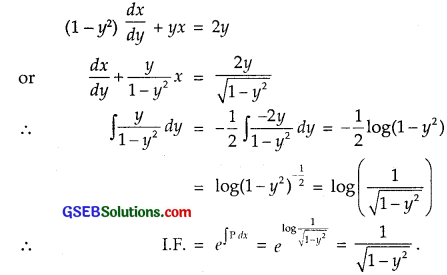∴ Part (D) is the correct answer.